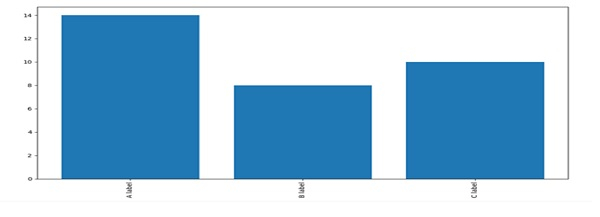# Barchart with vertical labels in Python/Matplotlib

First, we can create bars using plt.bar and using xticks. Then, we can align the labels by setting the “vertical” or “horizontal” attributes in the “rotation” key.

## Steps

• Make lists, bars_heights, and bars_label, with numbers.

• Make a bar plot using bar() method, with bars_heights and length of bars_label.

• Get or set the current tick locations and labels of the X-axis, using xticks() with rotation='vertical' and bars_label.

• To show the plot, use plt.show() method.

## Example

from matplotlib import pyplot as plt

bars_heights = [14, 8, 10]
bars_label = ["A label", "B label", "C label"]

plt.bar(range(len(bars_label)), bars_heights)
plt.xticks(range(len(bars_label)), bars_label, rotation='vertical')
plt.show()

## Output